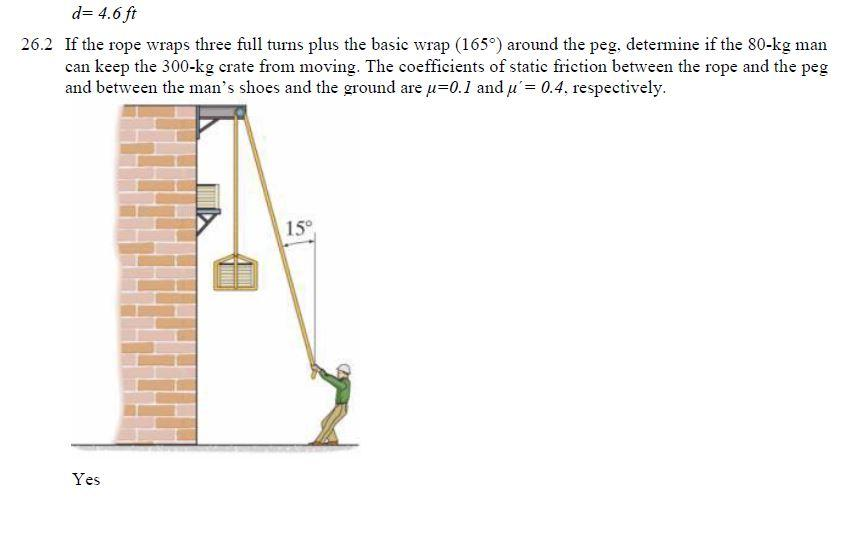# Question d= 4.6 ft 26.2 If the rope wraps three full turns plus the basic wrap (1659) around the peg, determine if the 80-kg man can keep the 300-kg crate from moving. The coefficients of static friction between the rope and the peg and between the man's shoes and the ground are u=0.1 and u'= 0.4. respectively. 15° YesRG78VD The Asker · Civil EngineeringTranscribed Image Text: d= 4.6 ft 26.2 If the rope wraps three full turns plus the basic wrap (1659) around the peg, determine if the 80-kg man can keep the 300-kg crate from moving. The coefficients of static friction between the rope and the peg and between the man's shoes and the ground are u=0.1 and u'= 0.4. respectively. 15° Yes
More
Transcribed Image Text: d= 4.6 ft 26.2 If the rope wraps three full turns plus the basic wrap (1659) around the peg, determine if the 80-kg man can keep the 300-kg crate from moving. The coefficients of static friction between the rope and the peg and between the man's shoes and the ground are u=0.1 and u'= 0.4. respectively. 15° Yes